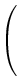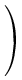Section 4.2 - Convolutions

First, some math:

I will try to explain clearly what a convolution products between two matrix works is :-)

First of all, a matrix is a kind of table of numbers. Example:1 0-2 4 3 0

This is a 3x2 matrix...

Let's first name the matrixes m1 and m2, and m is the destination.
Let's also give coordinates to both matrixes. So m1(2,3) is item of column two and row three.

We define the convolution product m = m1 (x) m2 this way:

m(x,y) = the sum of all products of the form [m1(x1,y1)*m2(x2,y2), where x1-x2 = x and y1-y2 = y]. Don't panic, you'll understand with examples ;-)

There is no function in picFX for convultion products, so you will have to do everything 'by hand' and writing unfinishing expressions ( :-( ). That function might come once, even if it causes problems to code ;-)

You usually will have a picture which will be the first matrix (m1) and a smaller one (m2) usually not overcoming size 3×3. The destination matrix m is the resulting picture.
If you try to do a convolution between two pictures or larger matrix, you will quickly be overwhelmed by the size of the expressions ;-)
We will in this section work on two projects: one source-image and a destination project. I will reference them as projects zero and one.
All three components will always have the same formulas (just replacing r by g and then b ;-), so I will only tell you the red one.

A general formula would be: r(1,x,y) = r(0,x,y)*m2(0,0)+ r(0,x+1,y)*m2(1,0)+ ... + r(0,x+l,y)*m2(l,0)+ r(0,x,y+1)*m2(0,1)+ ... + r(0,x+i,y+j)*m2(i,j) + ... r(0,x+l,y+n)*m2(l,n).
Here, 1 is dest. project and 0 is source. l and n are size of the m2 matrix. Note that you can of course remove some constant to each x and y, to avoid having the picture 'moving' to the lower right.

Ok, enough math for now ;-) let us go on with nice pictures :-)

Choose a colourful and contrasted picture for the first project (0), so that you can easily see where all parts have gone, and make the second picture the same size as the first one.

* Let's begin with the classic Blur effect.

 We will do with m2 = 1/31 1-> Here the sum of all values of the matrix is one ( 1/3(1+1+1+0)=3/3 ), so the luminance of the resulting picture will be similar to the source's. 1 0

All values are positive, and it is what we call 'high pass' matrixes, which usually blurs the picture. (As, for instance, a bright pixel would be "spread" in the neighbouring pixels)...

This yields to:
r(1,x,y)=(r(0,x,y)*1+r(0,x+1,y)*1+r(0,x,y+1)*1+r(0,x+1,y+1)*0)/3, and after simplifications:

r(1,x,y)=(r(0,x,y)+r(0,x+1,y)+r(0,x,y+1))/3

If you want some better effect, you can also add the lower-right pixel: r(0,x+1,y+1) (and replace /3 by /4).

And if you want to give some more weight to a pixel, multiply it by two or even three. Do not forget to adjust the divider at the end to the sum of all factors in order to keep the lightness.

You could for example give some horizontal blur (to give a movement effect):

m2 = 1/6 ( 3 2 1 )

and the expression gets:

r(1,x,y)=(3*r(0,x,y)+2*r(0,x+1,y)+r(0,x+2,y))/6

...

* Now to the (also classic) relief effect. Note that it may be better to first turn the picture to black and white before doing it. Just try and see.

The idea is to calculate the difference between the current pixel and the ones that are near it, with the following matrix (horizontal relief):

m2 = (1 -1). Two things to see here: First the sum is zero, so all values will be around zero. Let's add 128 to the final expression to correct this.
Secondly, there's a negative value and m2 is a 'low-pass' matrix, which sometimes raises the contrast of the picture

r(1,x,y)=r(0,x,y)-r(0,x+1,y)+128

To have two-dimensional relief, you add horizontal and vertical relief:

 m2 =2 -1-1 0

r(1,x,y)=r(0,x,y)*2+r(0,x+1,y)*(-1)+r(0,x,y+1)*(-1)+r(0,x+1,y+1)*0+128

r(1,x,y)=2*r(0,x,y)-r(0,x,y+1)-r(0,x+1,y)+128

I let you play with these values (e.g. try multiplying the expression (before +128) by a constant to increase/decrease the effect.
Just take care to set a floating point mode when doing this, and avoid overcoming 256 or going under zero, it wouldn't look nice...
(even if you can use the min()/max() functions, but in these case the relief will be somehow "wrong".

To see a picture with more contrast, you can somehow add to that 'relief' matrix the pixel itself , this will let you see better where colour changes, but plain areas will keep the same colour. It is quite a nice effect.

 m2 =3 -1+1 0=4 -1-1 -1 0 0 -1 -1

The sum of the terms here is one, so you won't need to add 128...

r(1,x,y)=((4*r(0,x,y)-r(0,x+1,y))-r(0,x,y+1))-r(0,x+1,y+1)

It is possible, as I said above, to have the origin (the term in bold) elsewhere:

 1/50 1 01 1 1 0 1 0

This matrix does a blurring in all directions.

(Actually, if you do many times convolution operations having the origin in the top left corner, your picture will slowly migrate to the bottom right, every time you make an effect...)

The corresponding equation is (note the '-1' when we are on the first line (y-1) or the first column (x-1)...):

r(1,x,y)=(r(0,x,y-1)+r(0,x-1,y)+r(0,x,y)+r(0,x+1,y)+r(0,x,y+1))/5

Index

Chapter four: Inter-referencing

4.1: Having fun with colours
4.2: Convolutions
4.3: Linear transformations